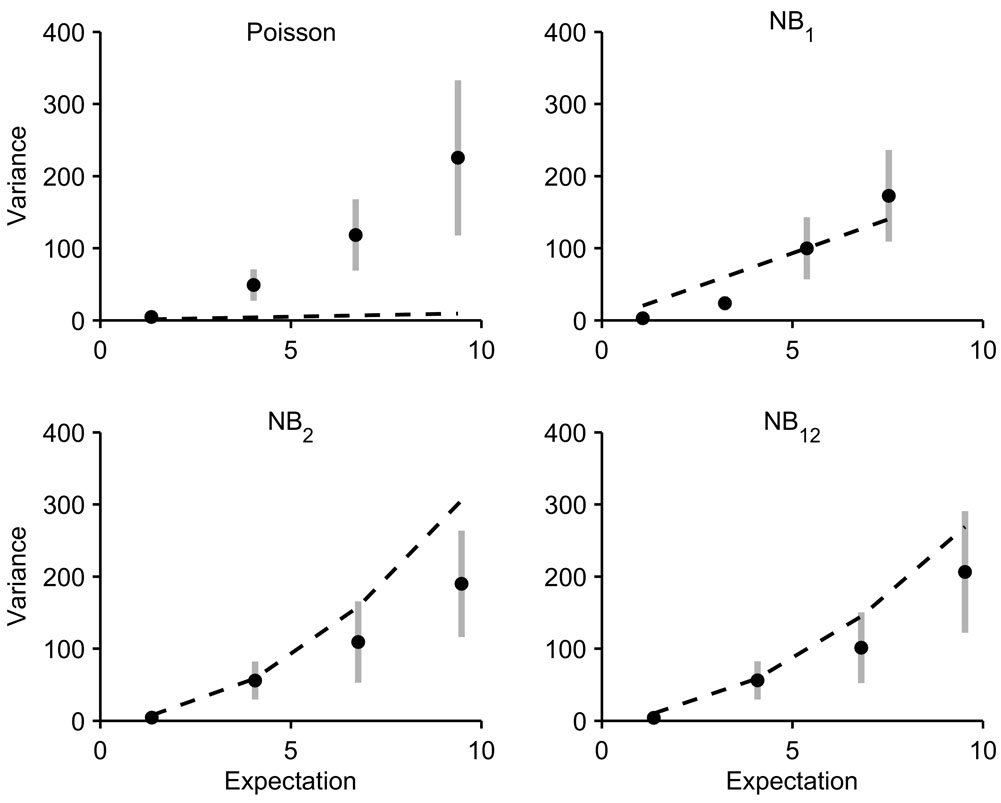### Andreas Lindén and Samu Mäntyniemi. 2011. Using the negative binomial distribution to model overdispersion in ecological count data. Ecology 92:1414–1421.

Appendix C. A figure with observed vs. fitted mean–variance relationships.FIG. C1. Mean–variance relationships based on four different hypotheses about overdispersion. The data are classified into five equally spaced groups according to the expected values of the models. The sample variances for each group (solid circles) are plotted against the class midpoints. The error bars span 2 standard errors in both directions (approximate 95% confidence intervals). The dashed lines show the mean–variance relationships assumed by the fitted models.

[Back to E092-120]